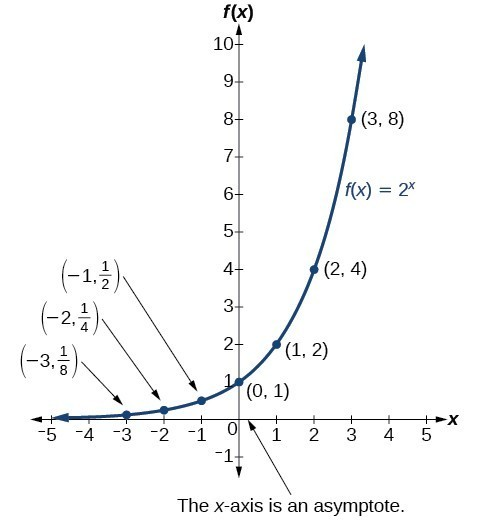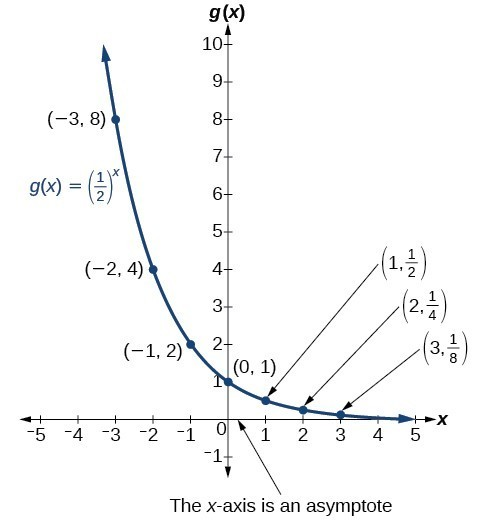## Characteristics of Graphs of Exponential Functions

### Learning Outcomes

• Determine whether an exponential function and its associated graph represents growth or decay.
• Sketch a graph of an exponential function.

Before we begin graphing, it is helpful to review the behavior of exponential growth. Recall the table of values for a function of the form $f\left(x\right)={b}^{x}$ whose base is greater than one. We’ll use the function $f\left(x\right)={2}^{x}$. Observe how the output values in the table below change as the input increases by 1.

 x –3 –2 –1 0 1 2 3 $f\left(x\right)={2}^{x}$ $\frac{1}{8}$ $\frac{1}{4}$ $\frac{1}{2}$ 1 2 4 8

Each output value is the product of the previous output and the base, 2. We call the base 2 the constant ratio. In fact, for any exponential function with the form $f\left(x\right)=a{b}^{x}$, b is the constant ratio of the function. This means that as the input increases by 1, the output value will be the product of the base and the previous output, regardless of the value of a.

Notice from the table that:

• the output values are positive for all values of x
• as x increases, the output values increase without bound
• as x decreases, the output values grow smaller, approaching zero

The graph below shows the exponential growth function $f\left(x\right)={2}^{x}$.Notice that the graph gets close to the x-axis but never touches it.

The domain of $f\left(x\right)={2}^{x}$ is all real numbers, the range is $\left(0,\infty \right)$, and the horizontal asymptote is $y=0$.

To get a sense of the behavior of exponential decay, we can create a table of values for a function of the form $f\left(x\right)={b}^{x}$ whose base is between zero and one. We’ll use the function $g\left(x\right)={\left(\frac{1}{2}\right)}^{x}$. Observe how the output values in the table below change as the input increases by 1.

 x –3 –2 –1 0 1 2 3 $g\left(x\right)=\left(\frac{1}{2}\right)^{x}$ 8 4 2 1 $\frac{1}{2}$ $\frac{1}{4}$ $\frac{1}{8}$

Again, because the input is increasing by 1, each output value is the product of the previous output and the base or constant ratio $\frac{1}{2}$.

Notice from the table that:

• the output values are positive for all values of x
• as x increases, the output values grow smaller, approaching zero
• as x decreases, the output values grow without bound

The graph below shows the exponential decay function, $g\left(x\right)={\left(\frac{1}{2}\right)}^{x}$.The domain of $g\left(x\right)={\left(\frac{1}{2}\right)}^{x}$ is all real numbers, the range is $\left(0,\infty \right)$, and the horizontal asymptote is $y=0$.

### A General Note: Characteristics of the Graph of the Parent Function $f\left(x\right)={b}^{x}$

An exponential function with the form $f\left(x\right)={b}^{x}$, $b>0$, $b\ne 1$, has these characteristics:

• one-to-one function
• horizontal asymptote: $y=0$
• domain: $\left(-\infty , \infty \right)$
• range: $\left(0,\infty \right)$
• x-intercept: none
• y-intercept: $\left(0,1\right)$
• increasing if $b>1$
• decreasing if $b<1$

### How To: Given an exponential function of the form $f\left(x\right)={b}^{x}$, graph the function

1. Create a table of points.
2. Plot at least 3 point from the table including the y-intercept $\left(0,1\right)$.
3. Draw a smooth curve through the points.
4. State the domain, $\left(-\infty ,\infty \right)$, the range, $\left(0,\infty \right)$, and the horizontal asymptote, $y=0$.

### Example: Sketching the Graph of an Exponential Function of the Form $f\left(x\right)={b}^{x}$

Sketch a graph of $f\left(x\right)={0.25}^{x}$. State the domain, range, and asymptote.

### Try It

Sketch the graph of $f\left(x\right)={4}^{x}$. State the domain, range, and asymptote.

## Contribute!

Did you have an idea for improving this content? We’d love your input.